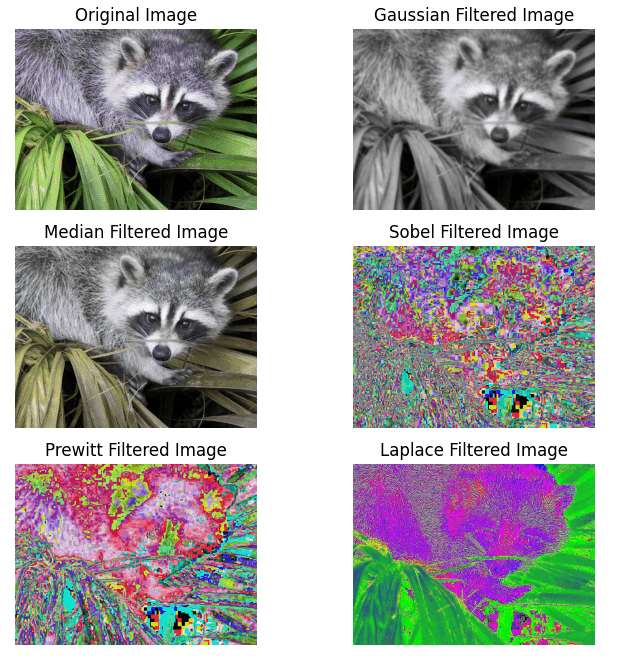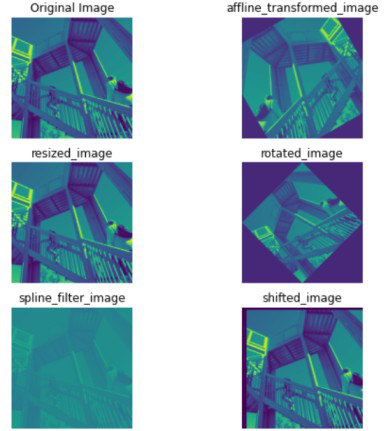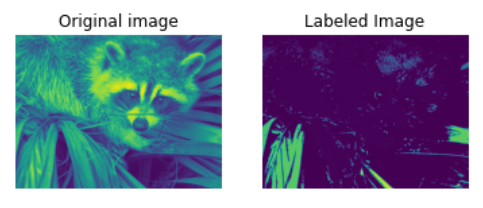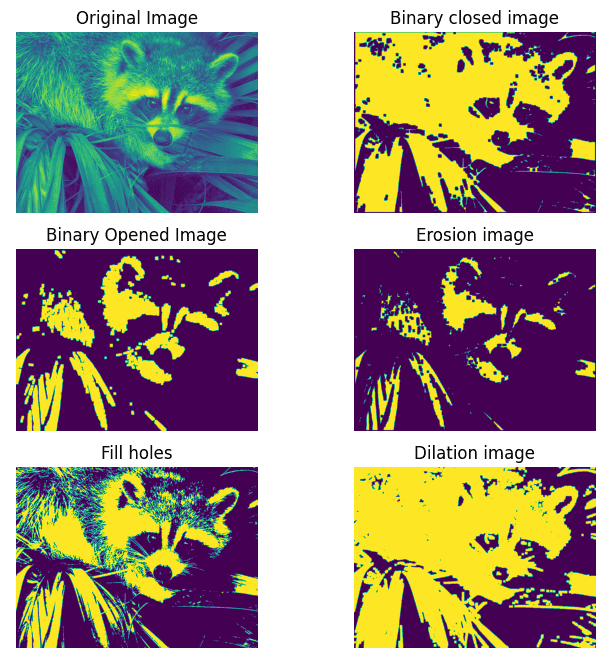Open in App
Not now

# Multidimensional image processing using Scipy in Python

• Last Updated : 30 Jan, 2023

SciPy is a Python library used for scientific and technical computing. It is built on top of NumPy, a library for efficient numerical computing, and provides many functions for working with arrays, numerical optimization, signal processing, and other common tasks in scientific computing.

Image processing is the field of computer science that deals with the manipulation, analysis, and interpretation of images. In image processing, a multidimensional image is an image that has more than two dimensions. This can include images with multiple color channels (e.g., red, green, and blue channels in a color image), images with multiple time points (e.g., a video), and images with multiple spatial dimensions (e.g., a 3D medical imaging scan).

SciPy provides several functions for processing multidimensional images, including functions for reading and writing images, image filtering, image warping, and image segmentation. The ‘scipy.ndimage’ is a module in the SciPy library that provides functions for multidimensional image processing. It is built on top of NumPy, a library for efficient numerical computing, and provides many functions for working with arrays, including functions for image processing.

Since there are a lot of operations possible, the article covers some of the basic operations

• Image filtering: We can use the scipy.ndimage.filters module to apply various types of image filters, such as median filters, Gaussian filters, and Sobel filters.
• Image interpolation: We can use the scipy.ndimage.interpolation module to warp images using various interpolation methods, such as nearest-neighbor, linear, and cubic.
• Image segmentation: We can use the scipy.ndimage.measurements module to perform image segmentation tasks, such as finding connected components and labeling objects in an image.
• Image manipulation: We can use the scipy.ndimage.morphology module to perform image manipulation tasks, such as erosion, dilation, and opening and closing.

### Image Filtering with SciPy

In image processing, filters are mathematical operations that are applied to an image to modify its appearance or extract specific features. Filters can be used for tasks such as smoothing, sharpening, enhancing edges, removing noise, and more. Many types of filters can be used in image processing, including linear filters, non-linear filters, and morphological filters. Linear filters apply a linear combination of the input image pixels to produce the output image, while non-linear filters apply a non-linear function to the input image pixels. Morphological filters are a type of non-linear filter that is based on the shape or structure of the image pixels.

The scipy.ndimage module in particular provides several filter functions for tasks such as smoothing, sharpening, and edge detection.

Here are some examples of filter functions that are provided by scipy.ndimage:

• Median filter: We can use the scipy.ndimage.filters.median_filter function to apply a median filter to an image, which can be used to remove noise from the image.
• Gaussian filter: We can use the scipy.ndimage.filters.gaussian_filter function to apply a Gaussian filter to an image, which can be used to smooth the image or reduce noise.
• Sobel filter: We can use the scipy.ndimage.filters.sobel function to apply a Sobel filter to an image, which is a type of edge detection filter that enhances edges in the image.
• Laplacian filter: We can use the scipy.ndimage.filters.laplace function to apply a Laplacian filter to an image, which is a second-order derivative filter that can be used to detect edges and corners in the picture.
• Prewitt filter: The Prewitt filter is a type of edge detection filter that is similar to the Sobel filter. It calculates the gradient of the image intensity and enhances edges in the image by identifying areas of rapid intensity change. We can use the scipy.ndimage.filters.prewitt function to apply a Prewitt filter to an image.

Here is a code snippet showing the implementation of all the filters.

## Python3

 `# Import the necessary libraries ` `import` `scipy.ndimage ` `from` `scipy ``import` `misc ` `import` `matplotlib.pyplot as plt ` ` `  `# reads a raccoon face ` `image ``=` `misc.face() ` `#image = plt.imread('Ganesh.jpg') ` ` `  `# Apply a Gaussian filter to the image ` `gaussian_filtered_image ``=` `scipy.ndimage.filters.gaussian_filter(image, sigma``=``3``) ` ` `  `# Apply Median Filter ` `median_filtered_image ``=` `scipy.ndimage.filters.median_filter(image, size``=``3``) ` ` `  `# Apply Sobel Filter ` `sobel_filtered_image ``=` `scipy.ndimage.filters.sobel(image) ` ` `  `# Apply Laplace Filter ` `laplace_filtered_image ``=` `scipy.ndimage.filters.laplace(image) ` ` `  `# Apply Prewitt Filter ` `prewitt_filtered_image ``=` `scipy.ndimage.filters.prewitt(image) ` ` `  ` `  `# Initialise the subplot function using number of rows and columns ` `figure, axis ``=` `plt.subplots(``3``, ``2``, figsize``=``(``8``, ``8``)) ` ` `  `# original image ` `axis[``0``, ``0``].imshow(image) ` `axis[``0``, ``0``].set_title(``"Original Image"``) ` `axis[``0``, ``0``].axis(``'off'``) ` ` `  `# gaussian filter ` `axis[``0``, ``1``].imshow(gaussian_filtered_image) ` `axis[``0``, ``1``].set_title(``"Gaussian Filtered Image"``) ` `axis[``0``, ``1``].axis(``'off'``) ` ` `  `# median filter ` `axis[``1``, ``0``].imshow(median_filtered_image) ` `axis[``1``, ``0``].set_title(``"Median Filtered Image"``) ` `axis[``1``, ``0``].axis(``'off'``) ` ` `  `# sobel filter ` `axis[``1``, ``1``].imshow(sobel_filtered_image) ` `axis[``1``, ``1``].set_title(``"Sobel Filtered Image"``) ` `axis[``1``, ``1``].axis(``'off'``) ` ` `  `# laplace filter ` `axis[``2``, ``1``].imshow(laplace_filtered_image) ` `axis[``2``, ``1``].set_title(``"Laplace Filtered Image"``) ` `axis[``2``, ``1``].axis(``'off'``) ` ` `  `# prewitt filter ` `axis[``2``, ``0``].imshow(prewitt_filtered_image) ` `axis[``2``, ``0``].set_title(``"Prewitt Filtered Image"``) ` `axis[``2``, ``0``].axis(``'off'``) ` ` `  `plt.show() `

Outputs:Image Filtering with SciPy

### Image Interpolation with scipy

Image interpolation is the process of estimating the values of pixels in an image based on the values of surrounding pixels. Image interpolation is often used to resize images or to correct distortions in images.

There are several different methods of interpolation that can be used for image processing, including nearest neighbor interpolation, bilinear interpolation, and bicubic interpolation. The choice of interpolation method can affect the quality and smoothness of the resulting image, as well as the computational complexity of the interpolation process.

Here are different types of interpolation transformations provided in the scipy.ndimage.

• Affine transformation:– An affine transformation is a linear transformation that preserves lines and parallelism. It is a type of transformation that can be used to rotate, scale, translate, or skew an image.
• Zoom:- The zoom function allows one to zoom in or out on an image by interpolating the values of the pixels. This can be useful for tasks such as image resizing or image analysis, where one may want to change the size of an image or examine the image at different scales.
• Shift:-  It allows to shift of an image by a specified amount. This can be useful for tasks such as image alignment or image analysis, where one may want to compare slightly offset images.
• Rotate:- It allows the rotation of an image by a specific amount. This can be useful for tasks such as image alignment or image analysis, where one may want to compare slightly offset images.
• Spline filter:- A spline filter is a type of smoothing filter that uses a spline function to fit a curve to the values of the pixels in the image. This can be useful for tasks such as image denoising or image smoothing, where you may want to reduce noise or smooth out rough edges in the image.

Here is a code snippet showing the implementation of all the Interpolation methods.

## Python3

 `# Import the necessary libraries ` `import` `scipy.ndimage ` `from` `scipy ``import` `misc ` `import` `numpy as np ` `import` `matplotlib.pyplot as plt ` ` `  `# reads a image ` `image ``=` `misc.ascent() ` ` `  `# apply Affline transformation ` `w, h ``=` `image.shape ` `# Rotate the image by 30° counter-clockwise. ` `theta ``=` `np.pi``/``6` ` `  `# first shift/center the image, apply rotation,and then apply inverse shift ` `mat_rotate ``=` `np.array( ` `    ``[[``1``, ``0``, w``/``2``], [``0``, ``1``, h``/``2``], [``0``, ``0``, ``1``]]) @ np.array( ` `    ``[[np.cos(theta), np.sin(theta), ``0``],  ` `     ``[np.sin(theta), ``-``np.cos(theta), ``0``], [``0``, ``0``, ``1``]]) @ np.array( ` `    ``[[``1``, ``0``, ``-``w``/``2``], [``0``, ``1``, ``-``h``/``2``], [``0``, ``0``, ``1``]]) ` ` `  `# affine transformation ` `affline_transformed_image ``=` `scipy.ndimage.affine_transform(image, mat_rotate) ` ` `  `# Resize the image using bilinear interpolation with zoom ` `resized_image ``=` `scipy.ndimage.interpolation.zoom(image, zoom``=``2``, order``=``1``) ` ` `  `# Shift the image by (10, 20) pixels ` `shifted_image ``=` `scipy.ndimage.interpolation.shift(image, shift``=``(``10``, ``20``)) ` ` `  `# Rotate the image by 45 degrees ` `rotated_image ``=` `scipy.ndimage.interpolation.rotate(image, angle``=``45``) ` ` `  `# Apply the spline filter to the image ` `filtered_image ``=` `scipy.ndimage.interpolation.spline_filter(image) ` ` `  ` `  `# Initialise the subplot function using number of rows and columns ` `figure, axis ``=` `plt.subplots(``3``, ``2``, figsize``=``(``8``, ``8``)) ` ` `  `# original image ` `axis[``0``, ``0``].imshow(image) ` `axis[``0``, ``0``].set_title(``"Original Image"``) ` `axis[``0``, ``0``].axis(``'off'``) ` ` `  `# affline transformation filter ` `axis[``0``, ``1``].imshow(affline_transformed_image) ` `axis[``0``, ``1``].set_title(``"affline_transformed_image"``) ` `axis[``0``, ``1``].axis(``'off'``) ` ` `  `# zoom filter ` `axis[``1``, ``0``].imshow(resized_image) ` `axis[``1``, ``0``].set_title(``"resized_image"``) ` `axis[``1``, ``0``].axis(``'off'``) ` ` `  `# rotate filter ` `axis[``1``, ``1``].imshow(rotated_image) ` `axis[``1``, ``1``].set_title(``"rotated_image"``) ` `axis[``1``, ``1``].axis(``'off'``) ` ` `  `# shift filter ` `axis[``2``, ``1``].imshow(shifted_image) ` `axis[``2``, ``1``].set_title(``"shifted_image"``) ` `axis[``2``, ``1``].axis(``'off'``) ` ` `  `# spline filter ` `axis[``2``, ``0``].imshow(filtered_image) ` `axis[``2``, ``0``].set_title(``"spline_filter_image"``) ` `axis[``2``, ``0``].axis(``'off'``) ` ` `  `plt.show() `

Output:Image Interpolation with scipy

### Image Segmentation using Scipy

Image segmentation is the process of dividing an image into different regions or segments based on specific criteria. It is a common technique used in image processing and computer vision applications to identify and extract objects or regions of interest in an image.

The scipy.ndimage.measurements module of the scipy library provides a number of functions that can be used for image segmentation. Here are a few examples:

• scipy.ndimage.measurements.label: This function allows you to label connected components in an image. It is often used as a preprocessing step for segmenting objects in an image.
• scipy.ndimage.measurements.mean: This function allows you to calculate the mean of the values of an image or array.
• scipy.ndimage.measurements.variance: This function allows you to calculate the variance of the values of an image or array.
• scipy.ndimage.measurements.standard_deviation: This function allows you to calculate the standard deviation of the values of an image or array.
• scipy.ndimage.measurements.center_of_mass: This function allows you to calculate the center of mass of an image or region of an image. It can be used to identify the locations of objects in an image.

Here is a code snippet showing the implementation of the label measurement function.

## Python3

 `#Import the necessary libraries ` `import` `scipy.ndimage ` `import` `numpy as np ` `import` `matplotlib.pyplot as plt ` `from` `scipy ``import` `misc ` ` `  `# Read the image ` `image ``=` `misc.face() ` ` `  `# Convert the image to grayscale ` `image ``=` `np.mean(image, axis``=``2``) ` ` `  `# Threshold the image to create a binary image ` `threshold ``=` `128` `binary_image ``=` `image > threshold ` ` `  `# Label the connected components in the binary image ` `labels, num_labels ``=` `scipy.ndimage.label(binary_image) ` ` `  `# plotting image ` `f ``=` `plt.figure() ` ` `  `# original image ` `f.add_subplot(``1``,``2``, ``1``) ` `plt.imshow(image) ` `plt.title(``'Original image'``) ` `plt.axis(``"off"``) ` ` `  `# labeled Image ` `f.add_subplot(``1``,``2``, ``2``) ` `plt.imshow(labels) ` `plt.title(``"Labeled Image"``) ` `plt.axis(``"off"``) ` ` `  `plt.show(block``=``True``)`

Output:Image Segmentation using Scipy

Implementation of several other measurement functionalities provided by the ndimage library are shown in the code below

## Python3

 `#Import the necessary libraries ` `import` `scipy.ndimage ` `import` `numpy as np ` `import` `matplotlib.pyplot as plt ` `from` `scipy ``import` `misc ` ` `  `# Read the image ` `image ``=` `misc.face() ` ` `  `# Convert the image to grayscale ` `image ``=` `np.mean(image, axis``=``2``) ` ` `  `# Threshold the image to create a binary image ` `threshold ``=` `128` `binary_image ``=` `image > threshold ` ` `  `# extrema ` `min_value, max_value, min_loc, max_loc ``=` `scipy.ndimage.extrema(image) ` ` `  `# mean value ` `mean ``=` `scipy.ndimage.mean(image) ` ` `  `# calculate center of mass ` `center_of_mass ``=` `scipy.ndimage.center_of_mass(image) ` ` `  `# standard deviation ` `standard_deviation``=` `scipy.ndimage.standard_deviation(image) ` ` `  `# variance ` `variance ``=` `scipy.ndimage.variance(image) ` ` `  `print``(``"Minimum pixel value in the image is {} on the location {}"``.``format``(min_value, min_loc)) ` `print``(``"Maximum pixel value in the image is {} on the location {}"``.``format``(max_value,max_loc)) ` `print``(``"Mean:- {} \nCenter of Mass:- {} \nStandard Deviation:- {} \nVariance:- {}"``.``format``( ` `    ``mean, ` `    ``center_of_mass, ` `    ``standard_deviation, ` `    ``variance))`

Output:-

```Minimum pixel value in the image is 0.3333333333333333 on the location (567, 258)
Maximum pixel value in the image is 254.0 on the location (245, 568)
Mean:- 110.16274388631183
Center of Mass:- (356.6752247435916, 469.240201083681)
Standard Deviation:- 54.975712030326186
Variance:- 3022.3289132413515```

### Image Manipulation with Scipy

The scipy.ndimage.morphology module of the scipy library provides a number of functions for performing morphological operations on images. Morphological operations are a set of image-processing techniques that involve the use of shapes or structuring elements to process images. These operations are often used for tasks such as image enhancement, noise reduction, and object extraction.

Here are a few examples of morphological operations that are available in scipy.ndimage.morphology:

• scipy.ndimage.morphology.binary_erosion: This function allows you to apply binary erosion to an image, which can be used to thin or remove small features from the image.
• scipy.ndimage.morphology.binary_dilation: This function allows you to apply binary dilation to an image, which can be used to thicken or add small features to the image.
• scipy.ndimage.morphology.binary_closing: This function allows you to apply morphological closing to an image, which can be used to fill small holes or remove small objects from the image.
• scipy.ndimage.morphology.binary_opening: This function allows you to apply morphological opening to an image, which can be used to remove small objects or fill small holes in the image.

The code snippet shows the working of all the above-mentioned functions

## Python3

 `#Import the necessary libraries ` `import` `scipy.ndimage ` `import` `numpy as np ` `import` `matplotlib.pyplot as plt ` `from` `scipy ``import` `misc ` ` `  `# Read the image ` `image ``=` `misc.face() ` ` `  `# Convert the image to grayscale ` `image ``=` `np.mean(image, axis``=``2``) ` ` `  `# Threshold the image to create a binary image ` `threshold ``=` `128` `binary_image ``=` `image > threshold ` ` `  `# Create a structuring element for the closing operation ` `structuring_element ``=` `np.ones((``10``, ``10``), dtype``=``np.bool_) ` ` `  `# Apply morphological closing to the binary image ` `closed_image ``=` `scipy.ndimage.morphology.binary_closing(binary_image, structure``=``structuring_element) ` ` `  `# Apply morphological opening to the binary image ` `opened_image ``=` `scipy.ndimage.morphology.binary_opening(binary_image, structure``=``structuring_element) ` ` `  `# Apply morphological binary erosion to the binary image ` `binary_erosion_image ``=` `scipy.ndimage.morphology.binary_erosion(binary_image, structure``=``structuring_element) ` ` `  `# Apply morphological binary dilation to the binary image ` `binary_dilation_image ``=` `scipy.ndimage.morphology.binary_dilation(binary_image, structure``=``structuring_element) ` ` `  `# Apply morphological binary fill holes to the binary image ` `binary_fill_holes ``=` `scipy.ndimage.binary_fill_holes(binary_image, structure``=``structuring_element) ` ` `  `# Initialise the subplot function using number of rows and columns ` `figure, axis ``=` `plt.subplots(``3``, ``2``, figsize ``=` `(``8``,``8``)) ` ` `  `# original image ` `axis[``0``, ``0``].imshow(image) ` `axis[``0``, ``0``].set_title(``"Original Image"``) ` `axis[``0``, ``0``].axis(``'off'``) ` ` `  `# closed_image ` `axis[``0``, ``1``].imshow(closed_image) ` `axis[``0``, ``1``].set_title(``"Binary closed image"``) ` `axis[``0``, ``1``].axis(``'off'``) ` ` `  `# Opened Image ` `axis[``1``, ``0``].imshow(opened_image) ` `axis[``1``, ``0``].set_title(``"Binary Opened Image"``) ` `axis[``1``, ``0``].axis(``'off'``) ` ` `  `# Erosion image ` `axis[``1``, ``1``].imshow(binary_erosion_image) ` `axis[``1``, ``1``].set_title(``"Erosion image"``) ` `axis[``1``, ``1``].axis(``'off'``) ` ` `  `# Dilation image ` `axis[``2``, ``1``].imshow(binary_dilation_image) ` `axis[``2``, ``1``].set_title(``"Dilation image"``) ` `axis[``2``, ``1``].axis(``'off'``) ` ` `  `# Fill holes  ` `axis[``2``, ``0``].imshow(binary_fill_holes ) ` `axis[``2``, ``0``].set_title(``"Fill holes"``) ` `axis[``2``, ``0``].axis(``'off'``) ` ` `  `plt.show()`

OutputImage Manipulation with Scipy

My Personal Notes arrow_drop_up
Related Articles